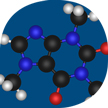# Independent Researcher: Ante Miličević

## Research

Research on the development of models for the prediction of stability constants of coordination compounds based on the molecular valence connectivity index 3χv.We are currently studying three basic problems in the prediction of stability constants: the choice of reliable experimental data for the calibration of the model, writing an appropriate formula (graph) of the complex, and development of a proper form of the regression function. In a paper we also made a statistical analysis of 14 experimental and seven theoretical values for the stability constant of copper(II) mono-complex with glycine and found that theoretical values are in no way worse than the experimental ones. In a lecture, it was shown that models could be much improved if resonant structures were taken into account.

Also, by way of a QSAR analysis A. Miličević joined a research group whose aim was to evaluate new oximes in creation of butyrylcholinesterase-based nerve agent bioscavenger. More than 1000 descriptors were tested, and for all data sets the most consistent models for pIC50 were obtained with the Eccentric Connectivity Index, ξc. The models yielded r = 0.870, 0.950, 0.952 and 0.957, respectively, for the first (N = 9), second (N=12), and third (N=13) subset, and complete set (N=34).

## Employees